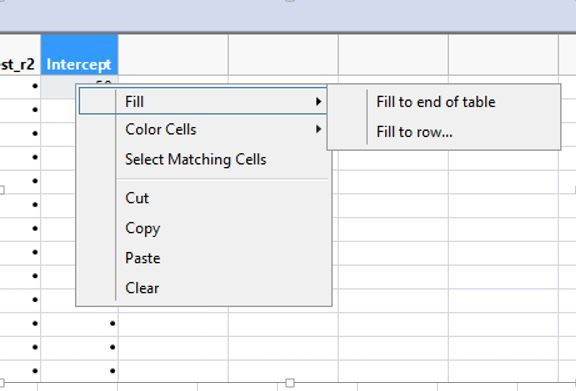Choose Language Hide Translation Bar
Highlighted

## JSL fill to end of table using first row value

Hi,

Using JSL script, I want the first cell value in "Intercept" column to Fill to end of table with the same value in first cell. Can anyone show me how to write it in script?1 ACCEPTED SOLUTION

Accepted Solutions
Highlighted

## Re: JSL fill to end of table using first row value

I think the answer depends on what else is happening within your JSL, and how you want a user (assuming there is one) to interact with this. You can do something like:

``````Names Default To Here( 1 );
dt = Open( "\$SAMPLE_DATA/Big Class.jmp" );
dt << New Column( "X" );
dt:X = 5;
dt << New Column( "X2", Formula(if(Row() == 1, :X, Lag(:X2, 1))));
dt << runFormulas;
Wait(2);
dt:X = 10;
dt << runFormulas;``````

but that doesn't seem very useful. Because a formula column is computed, JMP will not allow you to edit one of the cells therein.

4 REPLIES 4
Highlighted

## Re: JSL fill to end of table using first row value

Using 'Help > Scripting Index' is good for this kind of thing. In this case, the example is:

``````Names Default To Here( 1 );
dt = Open( "\$SAMPLE_DATA/Big Class.jmp" );
dt << New Column( "X" );
dt:X << Set Each Value( 5 );``````
Highlighted

## Re: JSL fill to end of table using first row value

Hi Ian,

What if my value in first row can change from time to time instead of having a fix value? Can I script it in a way that it will always use the first row value (not a fix value) to fill up the rest of the rows in that column?

Highlighted

## Re: JSL fill to end of table using first row value

I think the answer depends on what else is happening within your JSL, and how you want a user (assuming there is one) to interact with this. You can do something like:

``````Names Default To Here( 1 );
dt = Open( "\$SAMPLE_DATA/Big Class.jmp" );
dt << New Column( "X" );
dt:X = 5;
dt << New Column( "X2", Formula(if(Row() == 1, :X, Lag(:X2, 1))));
dt << runFormulas;
Wait(2);
dt:X = 10;
dt << runFormulas;``````

but that doesn't seem very useful. Because a formula column is computed, JMP will not allow you to edit one of the cells therein.

Highlighted

## Re: JSL fill to end of table using first row value

Ian is correct in his answer to your question.  I have at times wanted to do the OTHER thing in the menu image you showed (Fill to row ...), which is also pretty simple by just using the Row Index functions to select the cells you want to fill.

`dt << Select Where( Any( Row() == Index( 7, 10 ) ) );`

Article Labels

There are no labels assigned to this post.